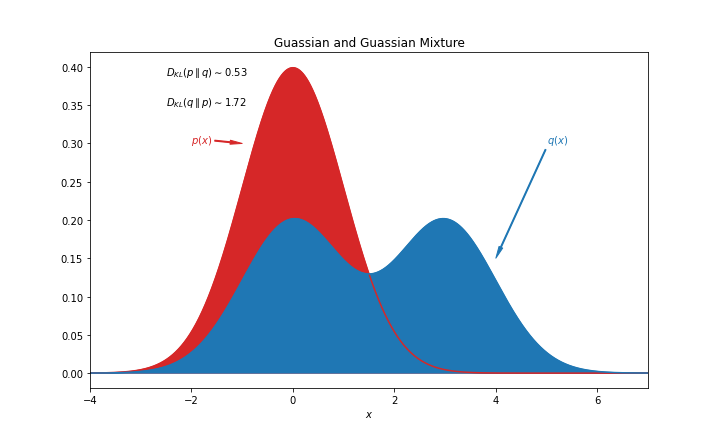# KL Divergence¶

The Kullback–Leibler (KL) divergence is defined as

\begin{align} \operatorname{D}_\mathrm{KL}(p \parallel q ) =& \mathbb E_{p} \left[\log\left(\frac{p}{q}\right) \right] \\ =& \int_{-\infty}^\infty p \log\left(\frac{p}{q}\right)\, dx . \end{align}

Suppose $$p$$ is a Gaussian distribution and $$q$$ is a bimodal Gaussian mixture, the KL divergence $$\operatorname{D}_\mathrm{KL}(p \parallel q )$$ and $$\operatorname{D}_\mathrm{KL}(q \parallel p )$$ are different as KL divergence is not necessarily symmetric. Thus the KL divergence is not a proper distance definition.KL divergence is a special case of f-divergence.

Contributors: LM